On-line GuidesAll GuideseBook StoreiOS / AndroidLinux for BeginnersOffice ProductivityLinux InstallationLinux SecurityLinux UtilitiesLinux VirtualizationLinux KernelSystem/Network AdminProgrammingScripting LanguagesDevelopment ToolsWeb DevelopmentGUI Toolkits/DesktopDatabasesMail SystemsopenSolarisEclipse DocumentationTechotopia.comVirtuatopia.comHow To GuidesVirtualizationGeneral System AdminLinux SecurityLinux FilesystemsWeb ServersGraphics & DesktopPC HardwareWindowsProblem SolutionsPrivacy Policy## Iteration Exercises

1. Greatest Common Divisor. The greatest common divisor is the largest number which will evenly divide two other numbers. Examples: GCD( 5, 10 ) = 5, the largest number that evenly divides 5 and 10. GCD( 21, 28 ) = 7, the largest number that divides 21 and 28.

GCD's are used to reduce fractions. Once you have the GCD of the numerator and denominator, they can both be divided by the GCD to reduce the fraction to simplest form. 21/28 reduces to 3/4.

Procedure 8.1. Greatest Common Divisor of two integers, p and q

1. Loop. Loop until p = q .

1. Swap. If p < q then swap p and q .

2. Subtract. If p > q then subtract q from p .

2. Result. Print p

2. Extracting the Square Root. This is a procedure for approximating the square root. It works by dividing the interval which contains the square root in half. Initially, we know the square root of the number is somewhere between 0 and the number. We locate a value in the middle of this interval and determine of the square root is more or less than this midpoint. We continually divide the intervals in half until we arrive at an interval which is small enough and contains the square root. If the interval is only 0.001 in width, then we have the square root accurate to 0.001

Procedure 8.2. Square Root of a number, n

1. Two Initial Guesses

g1 ← 0

g2 n

At this point, g1 × g1 n ≤ 0 ≤ g2 × g2 n

2. Loop. Loop until abs( g1 × g1 n n < 0.001

1. Midpoint.  mid ← ( g1 + g2 )÷2

2. Midpoint Squared vs. Number.  sqrt_mid mid × mid number

3. Which Interval?

if sqrt_mid ≤ 0 then g1 mid

if sqrt_mid ≥ 0 then g2 mid

if sqrt_mid is zero, mid is the exact answer!

3. Result. Print g1

3. Sort Four Numbers. This is a challenging exercise in if-statement construction. For some additional insight, see [Dijkstra76], page 61.

Given 4 numbers ( W , X , Y , Z )

Assign variables `w`, `x`, `y`, `z` so that `w``x``y``z` and `w`, `x`, `y`, `z` are from W , X , Y , and Z . Do not use an array. One way to guarantee the second part of the above is to initialize `w`, `x`, `y`, `z` to W , X , Y , Z , and then use swapping to rearrange the variables.

Hint: There are only a limited combination of out-of-order conditions among four variables. You can design a sequence of if statements, each of which fixes one of the out-of-order conditions. This sequence of if statements can be put into a loop. Once all of the out-of-order conditions are fixed, the numbers are in order, the loop can end.

4. Highest Power of 2. This can be used to determine how many bits are required to represent a number. We want the highest power of 2 which is less than or equal to our target number. For example 64 ≤ 100 < 128. The highest power of 2 ≤ 100 is 26.

Given a number n , find a number p such that 2 p n < 2 p +1.

This can be done with only addition and multiplication by 2. Multiplication by 2, but the way, can be done with the << shift operator. Do not use the `pow` function, or even the ** operator, as these are too slow for our purposes.

Consider using a variable c , which you keep equal to 2 p . An initialization might be p ←1, c ←2. When you increment p by 1, you also double c .

Develop your own loop. This is actually quite challenging, even though the resulting program is tiny. For additional insight, see [Gries81], page 147.

5. How Much Effort to Produce Software? The following equations are the basic COCOMO estimating model, described in [Boehm81]. The input, K , is the number of 1000's of lines of source, total source lines÷1000.

Equation 8.1. Development Effort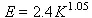Equation 8.2. Development Cost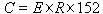Equation 8.3. Project Duration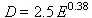Equation 8.4. Staffing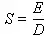Where K is the number of 1000's of lines of source, E is effort in staff-months, D is duration in calendar months, S is the average staff size, R is the billing rate, C is the cost in dollars (assuming R \$ per hour, and 152 working hours per staff-month).

Evaluate these functions for projects which range in size from 8,000 lines ( K =8) to 64,000 lines ( K =64) in steps of 8. Produce a table with lines of source, Effort, Duration, Cost and Staff size.

6. Wind Chill Table. Wind chill is used by meteorologists to describe the effect of cold and wind combined. Given the wind speed in miles per hour, v , and the temperature in °F, t , the Wind Chill, w , is given by the formula below. See Wind Chill in the section called “Numeric Types and Expressions” for more information.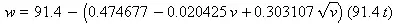Wind speeds are for 0 to 40 mph, above 40, the difference in wind speed doesn't have much practical impact on how cold you feel.

Evaluate this for all values of v (wind speed) from 0 to 40 mph in steps of 5, and all values of t (temperature) from -10 to 40 in steps of 5.

7. Celsius to Fahrenheit Conversion Tables. We'll make two slightly different conversion tables.

For values of Celsius from -20 to +30 in steps of 5, produce the equivalent Fahrenheit temperature. The following formula converts C (Celsius) to F (Fahrenheit).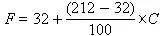For values of Fahrenheit from -10 to 100 in steps of 5, produce the equivalent Celsius temperatures. The following formula converts F (Fahrenheit) to C (Celsius).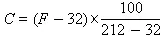8. Dive Planning Table. Given a surface air consumption rate, c , and the starting, s , and final, f , pressure in the air tank, a diver can determine maximum depths and times for a dive. For more information, see Surface Air Consumption Rate in the section called “Numeric Types and Expressions”.

Accept c , s and f from input, then evaluate the following for d from 30 to 120 in steps of 10. Print a table of t and d .

For each diver, c is pretty constant, and can be anywhere from 10 to 20, use 15 for this example. Also, s and f depend on the tank used, typical values are s =2500 and f =500.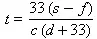9. Computing π. Each of the following series compute increasingly accurate values of π (3.1415926...)

•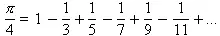•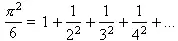•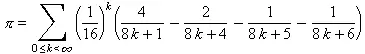•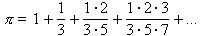10. Computing e. A logarithm is a power of some base. When we use logarithms, we can effectively multiply numbers using addition, and raise to powers using multiplication. Two Python built-in functions are related to this: `math.log` and `math.exp`. Both of these compute what are called natural logarithms, that is, logarithms where the base is e . This constant, e , is available in the math module, and it has the following formal definition:

Equation 8.5. Definition of e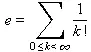For more information on the Σ operator, see the section called “Digression on The Sigma Operator”.

Equation 8.6. Definition of Factorial, n!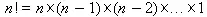4!=4×3×2×1. By definition, 0! = 1.

If we add up the values 1/0! + 1/1! + 1/2! + 1/3! + 1/4! + ... we get the value of e. Clearly, when we get to about 1/10!, the fraction is so small it doesn't contribute much to the total.

We can do this with two loops, an outer loop to sum up the 1/ k !'s, and an inner loop to compute the k !'s.

However, if we have a temporary value of k !, each time through the loop we can multiply the temporary by k , and then add 1/ temp to the sum.

You can check your work against `math.e` ~ 2.71828... or ```math.exp ```( `1` ).

11. Hailstone Numbers. For additional information, see [Banks02].

Start with a small number, n , 1 ≤ n ≤ 30.

There are two transformation rules that we will use:

• If n is odd, multiple by 3 and add 1 to create a new value for n .
• If n is even, divide by 2 to create a new value for n .

Perform a loop with these two transformation rules until you get to n = 1. You'll note that when n = 1, you get a repeating sequence of 1, 4, 2, 1, 4, 2, ...

You can test for oddness using the % (remainder) operation. If `n % 2 == 1`, the number is odd, otherwise it is even.

The two interesting facts are the “path length”, the number of steps until you get to 1, and the maximum value found during the process.

Tabulate the path lengths and maximum values for numbers 1..30. You'll need an outer loop that ranges from 1 to 30. You'll need an inner loop to perform the two steps for computing a new n until n == 1; this inner loop will also count the number of steps and accumulate the maximum value seen during the process.

Check: for 27, the path length is 111, and the maximum value is 9232.Published under the terms of the Open Publication License Design by Interspire